CipherJan 26, 2017

Cargo Ship Departure from Space Station, weak NASA coding as always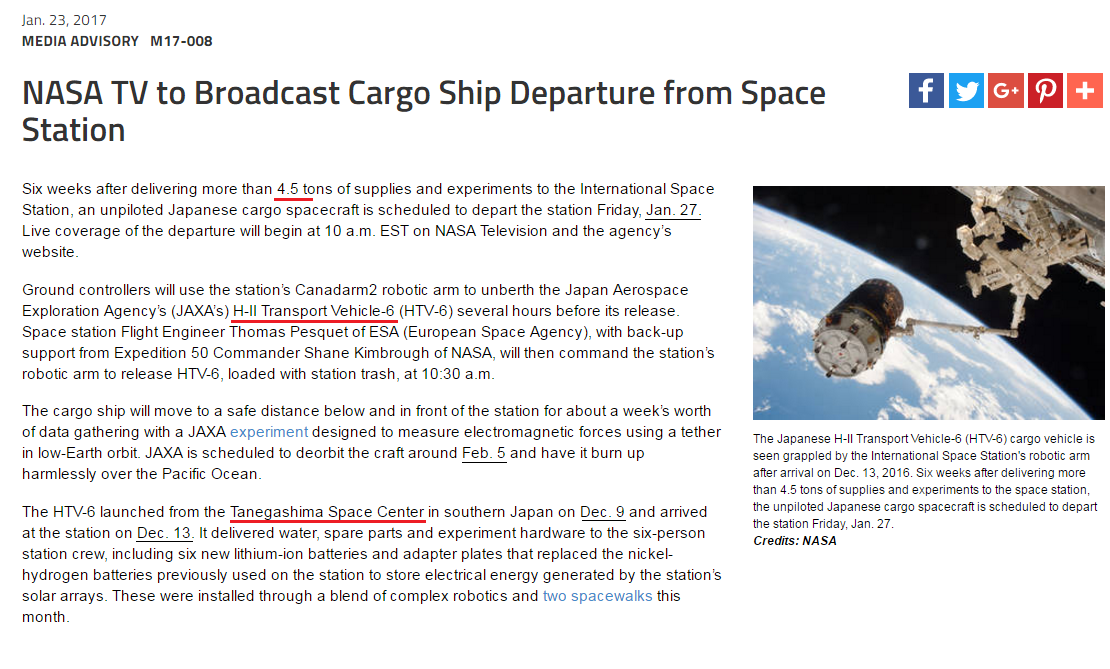"Six weeks after delivering more than 4.5 tons of supplies and experiments to the International Space Station"
International Space station = 9+5+2+5+9+5+1+2+9+6+5+1+3 + 10+7+1+3+5 + 10+2+1+2+9+6+5 = 123 (S Exception)
H-II Transport Vehicle-6 = 8+9+9 + 2+9+1+5+1+7+6+9+2 + 22+5+8+9+3+3+5 = 123 (K/V Exception)
Conspiracy = 3+15+14+19+16+9+18+1+3+25 = 123 (Ordinal)
H-II Transport Vehicle-Six = 8+9+9 + 100+80+1+40+90+60+50+80+100 + 700+5+8+9+3+20+5+90+9+300 = 1776 (Jewish)
International Space station = 9+40+100+5+80+40+1+100+9+50+40+1+20 + 90+60+1+3+5 + 90+100+1+100+9+50+40 = 1044 (Jewish)
They always make sure to make up precisely everything by the numbers, NASA is surely one of the easiest organizations to decode

Six weeks = 19+9+24 + 23+5+5+11+19 = 115 (Ordinal)
From December 9th when this cargo ship 'launched' to the end of the mission on February 5th is 58 days
February Fifth = 6+5+2+80+200+1+80+400 + 6+9+6+100+8 = 903 (Jewish)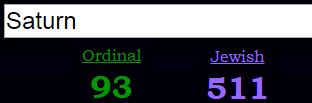Fifty eight = 6+9+6+20+25 + 5+9+7+8+20 = 115 (Ordinal)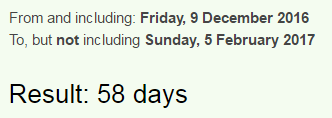December Ninth = 4+5+3+5+4+2+5+9 + 5+9+5+2+8 = 66 (Reduced)
Thirty-three = 2+8+9+9+2+7+2+8+9+5+5 = 66 (Reduced)

From arrival of cargo ship to the 'space station' on  December 13th to the release of this post is 42 days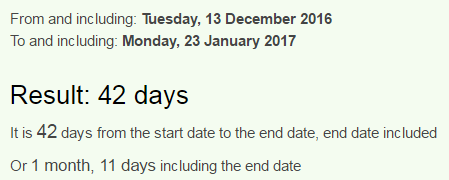The vehicle is run by JAXA (Japan Aerospace Exploration Agency)
Japan = 10+1+16+1+14 = 42 (Ordinal)
Commander Shane Kimbrough = 3+6+4+4+1+5+4+5+9 + 1+8+1+5+5 + 2+9+4+2+9+6+3+7+8 = 111 (Reduced)

December 13th and 1/23/2017 have exactly the same date numerology
12/13/2016 - 1+2+1+3+2+0+1+6 = 16 (1/23/2017 - 1+2+3+2+0+1+7 = 16)
Sixteen = 1+9+6+2+5+5+5 = 33 (Reduced)
12/13/16 - 12+13+16 = 41 (1/23/17 - 1+23+17 = 41) - 13th prime number is 41, also if we don't count the end date when the news is released, we have 41 days from the time it 'arrived to the space station'

It was launched from 'Tanegashima Space Center'
'Tanegashima Space Center' = 2+1+5+5+7+1+1+8+9+4+1 + 1+7+1+3+5 + 3+5+5+2+5+9 = 90 (Reduced)
'Japanese cargo spacecraft' = 1+1+7+1+5+5+1+5 + 3+1+9+7+6 + 1+7+1+3+5+3+9+1+6+2 = 90 (Reduced)
Ninety = 5+9+5+5+2+7 = 33 (Reduced)

They 'delivered' more than 4.5 tons

From the launch of cargo ship to the release of this post is 45 daysAlso from the time it 'arrived' to the 'space station' to departure from space station is 45 days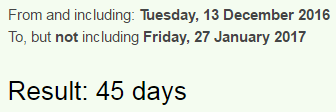45 is associated with the magic square of Saturn and also the 'scientists say that Saturn is 4.5 billion years old.. All of the NASA works end up going to Saturn really

Also I just found the first thing written out that has the same ordinal and reverse ordinal gematria
One seven seven six = 15+14+5 + 19+5+22+5+14 + 19+5+22+5+14 + 19+9+24 = 216 (Ordinal)
One seven seven six = 12+13+22 + 8+22+5+22+13 + 8+22+5+22+13 + 8+18+3 = 216 (Reverse Ordinal)